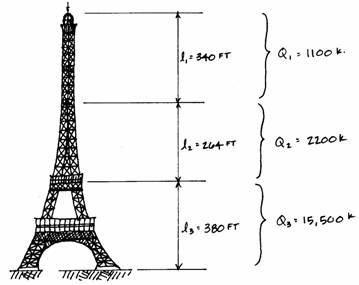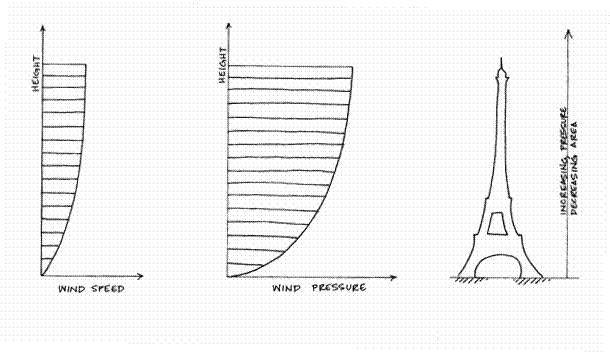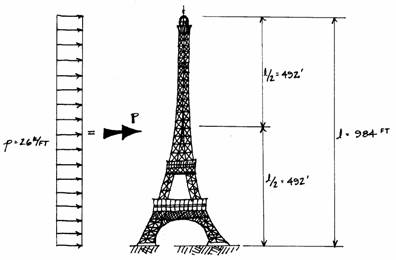Three types of loads act on the Eiffel Tower:

·        Dead loadits own weight·        Live loadthe weight of people and machinery on the platformsThe weight of the Tower is known from documents to be 18,800 kips.  This weight is not distributed uniformly along the height of the Tower; there is more material at the base than near the top.  A detailed analysis would divide this weight among many parts of the Tower, but for our purposes, the weight will be divided among the three portions defined earlier.  Estimates of these weights are shown below:The live load of 50 pounds per square foot is calculated over the two lower platforms where it is most significant.  The first platform is 240 square feet and the second platform is 110 square feet.  The total live load is computed from the load on each of these platforms:This load acts through the centroid of the Tower, which is located 257 feet above the ground.

The wind pressure on the Tower is stronger near the top than at the bottom, but the wind force is fairly uniform because the Tower is tapered.This analysis will use the assumption that the wind is a uniform load acting all along the Tower, but a conservatively high force is used to simulate high wind speeds.  This value is taken as p = 2.6 k/ft.  A more refined analysis would account for the variable force of the wind along with the diminishing area of the Tower.  Realizing that the assumptions may produce imprecise results (but still good estimates) one can find the total force from the wind.  Along the height of 984 feet, the total force is:The centroid of this force is halfway up the Tower so P, the idealized point wind load, acts at this point.  This is illustrated in the following diagram:Structural Studies Home